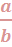# Unit 3

• Ratios, Rates, Unit Rates, Proportions, Percents

### Ratios

A ratio is a comparison of two things. We might compare boys to girls, cars to trucks or hours asleep to hours awake.
Whatever we choose to compare can then be written as a ratio. There are three different forms.  A ratio can be written as a to b, a : b orRatios can be part-to-part, part-to-whole, or whole-to-part.   For more on ratios, visit https://www.mathsisfun.com/numbers/ratio.html

Quick game to help students recognize ratios:  http://www.mathplayground.com/ASB_RatioMartian.html

• ### Unit Rate

rate is a comparison of two measurements or amounts that have different units. For example, 60 miles in 2 hours is a rate. A unit rate is a rate in which the second rate is 1 unit. For example, 30 miles in 1 hour, or 30 miles per hour, is a unit rate.

https://www.brainingcamp.com/lessons/rates/

• ### Proportions

proportion is an equation stating that two ratios are equivalent.

4 dogs:1 cat = 12 dogs: 3 cats is a proportion.

We usually write proportions in fraction form because it is easier to work with them that way.We can use Cross Multiplication to solve a missing part of a proportion:Multiply across the known corners,  then divide by the third number.  And we get this:  (42 × 10) / 20 = 420 / 20 = 21

Mathisfun https://www.mathsisfun.com/algebra/proportions.html

Proportions Dirt Bike Racing Game: http://www.mathplayground.com/ASB_DirtBikeProportions.html